# Sets Solutions

Here are the detailed explanations of the Sets Questions. The solutions are easily understandable so that the candidates can easily learn the topic and ace their CAT exam.

#### Test Your Sets & Venn Diagrams Basics Skills-Solutions

1. Option (d)

S = 110+80+120+70 = 380; X needs to be maximized.

I = 80.

S = I + 2II + 3III + 4 IV= 380.

To maximize X one needs to minimize the overlapped regions.

Assume III= 0, IV = 0.

So that 2II = 380-80 = 300. II = 150. X max = 80 + 150 = 230.

2. Option (c)

Given that S= 310 (i.e.100+ 70+ 140).

II +3III= 40+30+60 = 130

III=10 =>II = 100

S-X=II+2III

X=310-120= 190 => No. of candidates having none of the three = 200-190=10.

3. Option (c)

S = 25+60+50=135

X=100 (given)

S-X= II+2III

35= II+2III

The least value II can take is 1, as the number of people has to be a whole number

2III= 34 =>III(max) = 17.

4. Option (d)

In this case, the minima of all is that part of the venn diagram which has 5 overlapping regions. This can be found using a shortcut technique.

To find the minimum number of spectators who are fans of all 5 teams, take the difference of each from X (i.e.the value of atleast 1), add it up and call the sum Y; then take the difference of Y from 100 again to get the value Here X=100,

Step 1- Taking the difference of each from X

100-90=10

100-85=15

100-70=30

100-75=25

100-80=20

Total(Y) ∑=100

Step 2- Take the difference of Y from 100, 100-100=0 Minimum number of spectators who are fans of all 5 teams = 0.

5. Option (b)

S= 90+80+80=250

X= 100

Using the Maxima of all shortcut

(S-X)/(N-1) = 150/2= 75.

6. Option (b)

Using the venn diagram approach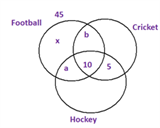II +III= 35,

a+b=20

Hence percentage playing only Football = 45-10-20 =15%.

Questions 7 & 8:

Soln: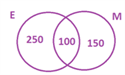n (E + M) = n(E) + n(M) – n(E ∩ M)

500 = 350 + 250 – n (E ∩ M)

n (E ∩ M) = 100

7. Option (a)

Since100 are both Engineers and MBAs.

8. Option (d)

People who are only Engineer = 250

People who are only MBA = 150

No. of people who are either only MBA or only Engineer = 250 + 150 = 400

9. Option (a)

X = I + II + III = 200

S = I + 2II + 3III = 140 + 150 + 160 = 450

S – X = II + 2III = 450 – 200 = 250

For III to be the minimum, II has to be the maximum. Now, II can take the maximum value of 200.

So, minimum value of III = 250 – 200= 50.

Questions 10 & 11: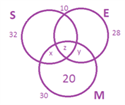Consider Maths:

It is given that 20 students failed only in Maths.

So, x + z + y + 20 = 30 ➜x + y + z = 10

Also x + z = 8 y + z = 6 x + y + 2 z = 14 ➜ z = 14 – 10 = 4, then x = 4 and y = 2

So, redrawing the Venn diagram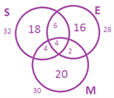10. Option (d)

Number of students who appeared in the examination = 18 + 20 + 16 + 6 + 4 + 2 + 4 = 70.

11. Option (d)

The number of students who failed in English and Science but not Mathematics = 6.

Questions 12-14: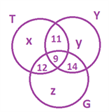Number of students = 278 = x + y + z + 11 + 12 + 14 + 9

Let us consider the total number of seats: x + y + z + 2(11 + 12 + 14) +

(3×9) = [x + y + z + 11 + 12 + 14 + 9] + [11 + 12 + 14 + 9 + 9] = 278 + 55 = 333.

So, number of seats in each class = 333/3 = 111

x = 111 – (11 + 9 + 12) = 79

z = 111 – (12 + 9 + 14) = 76

y = 111 – (11 + 9 + 14) = 77

12. Option (a)

No. of students who have occupied only one seat = x + y + z = 79 + 76 + 77 = 232.

13. Option (d)

No. of students who have occupied seat in Violin Class or Guitar Class, but not in Tabla Class = 77 + 14 + 76 = 167.

14. Option (b)

No. of students who have occupied a seat in Tabla and Violin Class, but not in Guitar Class = 20 – 9 = 11.

Questions 15-17:

18 married workers are engineers out of which

9 are men ➜ s + q = 18,

s = 9 ➜ q = 9

12 men are engineers ➜ r = 12.

12 men are married ➜ p = 12.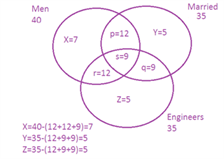15. Option (d)

No. of unmarried engineer in the company = 5 + 12 = 17.

16. Option (b)

No. of unmarried women = 5 {unmarried women: not men + not married i.e. z).

17. Option (a)

No. of engineer men not married = r = 12.

Questions 18-20:

From the data given in the question, one can fill up the Venn diagram as follows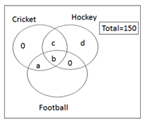From the information given, a+b+c+d=60, b =0.

18. Option (c)

The number of people who opted for only football= 150-60=90.

19. Option (d)

The number of people who opted only for hockey cannot be determined (d).

20. Option (c)

The number of people who opted for only football and only cricket = 0+90=90.

21. Option (c)

x- number who play all 3 games

80= 125 –(14+12+20) +x

x= 1, S=125, X=80

S-X=45=II+2III II+III=46.

So III=1 The Venn diagram can be represented as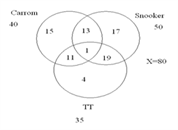22. Option (c)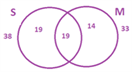So, the % of students failed in at least one subject = 19 + 19 + 14 = 52 %

So, the % of students who didn’t fail even in one subject = 100 – 52 = 48% % of students failed in Science = 38%  % of students who passed in Science = 100 – 38 = 62% % of students failed in Maths = 33%  % of students who passed in Mathematics = 100 – 33 = 67% Now, consider the % of students who passed: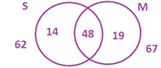So, as we see from the Venn diagram, % of students passed in only Science = 14%. If the total no. of students who appeared = x, then .14x = 700  x = 5000.

23. Option (a)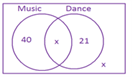be odd always .option c and d is out Hence x= 2. option a = 65 is possible.

24. Option (b)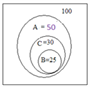From the Venn diagram given, it’s clear that no. of people who like C and not B are 30 – 25 = 5. People who like D are 100 – 50 = 50. Therefore, 5/50× 100 = 10%.

25. Option (d)

Since the question is about percentages, assume a base value 100 (total number of volunteers)

No. of volunteers receiving < 6 hours of sleep = 80

No. of volunteers who receive > 6 hours of sleep = 20 70 % of the above report no feeling of tiredness =14

Number of volunteers with > 6 hours of sleep and report tired = 6

Volunteers who receive < 6 hours of sleep and report feeling tired = 75

Number of volunteers who receive < 6 hours of sleep and are not tired = 80-75= 5

Total percentage of volunteers who report no feeling of tiredness during shifts = 14+5 = 19%.

So, the total percentage of volunteers who report no feeling of tiredness during shifts = 14+5 = 19%.

Stay tuned with Byju’s to get more important CAT Practice Questions with detailed solutions. Also, get updated with the latest CAT syllabus and pattern to prepare properly for the exam.